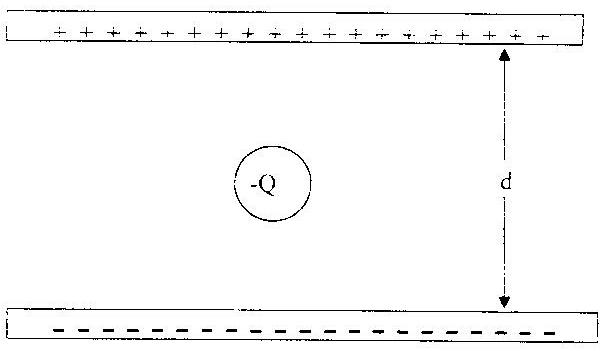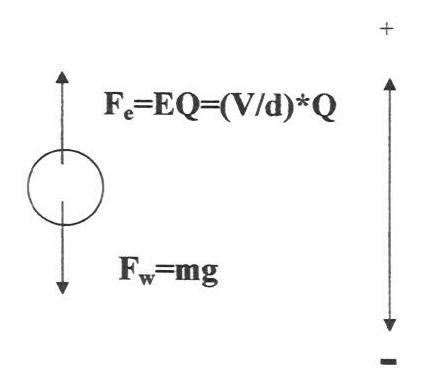Summer Research Program for Secondary School Teachers

Thomas Byrne

New Rochelle High School, Westchester

2002

The Elementary Charge of an Electron

Aim/Problem:

1.   How did Robert Millikan determine the elementary charge?

2.   Can we determine the mass of a single marble by measuring the mass of samples containing unknown numbers of identical marbles?

Objectives: The student will be able to:

1.   Diagram the forces acting on an object electrostatically attracted to the ceiling/wall of the classroom.

2.   Recall the relationship between electric force, electric field intensity and electric charge, as well as the relationship between electric field intensity, potential difference and distance between the parallel plates of a capacitor.

3.   Work cooperatively to devise an appropriate procedure for determining the mass of a single marble from the measurement of the mass of numerous samples containing unknown numbers of identical marbles.

4.   Extend that procedure to explain how MIllikan calculated the charge on a single electron from the measurement of the charges on numerous oil drops.

Materials:

-    Marbles that have been massed by the teacher and determined to have the same mass

-    Envelopes to be used to hold different numbers of marbles (between 12 and 30)

-    Mass of empty envelopes must be measured and recorded on each envelope

-    Triple beam balances for each student group

-    Data sheet to aid student organization of data (optional)

-    Ballons to be used as demonstration of oil drop

-    Model or diagram of apparatus used by Millikan in oil drop experiment

Plan:

1.   Begin with a discussion of why a balloon that is not charged by friction does not stick to the ceiling/wall while a balloon that was rubbed against clothing does stick:

-    Transfer of electrons cause balloon to become charged

-    Likely charge on the balloon (positive or negative)

-    How we could determine the number of electrons transferred (need to know the charge on the balloon and the charge on an electron)

-    Forces acting on balloon (electrostatic, gravity; drag and bouyant forces can be omitted in most classes)

Content Standards: 9-12

Standard B, Physical Science – Students develop an understanding of the components of atoms, and the electrical forces between charged objects.

6.   Review and diagram on the board the basic apparatus used by Millikan:

Parallel conducting plates and charged oil drops suspended between plates (use a balloon stuck to the board between two oppositely charged plates drawn on the board)The plates are hooked up to an adjustable voltage so that the field intensity between the plates can be adjusted: E = V/d.

Content Standards: 9-12

Standard B, Physical Science – Students develop an understanding that then electric force is a universal force that exists between any two objects and that opposite charges attract while like charges repel.

7.   Have students diagram and write equations for the forces acting on the oil drop (balloon) suspended between the plates:Content Standards: 9-12

Standard B, Physical Science – Students develop an understanding of gravitational and electrical forces.

8.   Discuss how we would write an equation for the condition of equilibrium of the oil drop and how we would manipulate the equation to solve for Q, the charge on the oil drop. (The students must assume the mass of the oil drop can be determined so that Q is the only unknown.)

Fe = Fw

(V/d)*Q = mg

Q = mgd/V

Content Standards: 9-12

Standard B, Physical Science – Students develop an understanding that objects change motion only when a net force is applied.

9.   Students divide into small groups. Each group will devise a strategy for solving a problem analagous to the work done by Millikan in determining the charge on a single electron:

Assignment : determine the mass of a single marble. The envelopes contain unknown numbers of identical marbles. Example, one envelope contains 12 marbles, another contains 15 and another 22. There should be at least 10 to 15 envelopes, with each envelope having its mass (i.e., the mass of the empty envelope) indicated on the front. Students may not open the envelopes or try to count the number of marbles inside by other means.

Teacher must monitor progress of groups and consider proposing questions to assist groups to develop a plan. Students must measure the mass of all or most of the samples and organize their data in a meaningful way. Teacher might provide a data sheet to assist in the process of organization of data.

Basic approach: Measure the gross mass of each envelope, substract the mass of the envelope to get the mass of the marbles in the envelope only. Organize the masses from lowest to highest. Get the difference between each of the masses. See if the lowest difference might be the mass of a single marble. Otherwise, perhaps guess and check, or try some other method that will result in the mass of a single marble.

KEY IDEA: Students should understand that after calculating the “net mass” of the marbles only in each envelope, the mass of a single marble must be a number that divides into each “net mass” so that the result is an integer.

Teaching Standards

Standard B – Students are given the opportunity to take responsibility for their own learning. Students work in small groups so that all participate in collecting and sharing data.

Standard D – Students are provided with resources, space and time to engage in inquiry-based tasks.

Standard E – Students are given the opportunity to collaborate with classmates and are required to explain and justify their understanding.

Content Standards: 9-12

Standard A, Science as Inquiry – Students identify questions and concepts that guide scientific investigations, use technology and mathematics to improve investigations and communications, formulate and revise scientific explanations and models using logic and evidence.

10. Relate the problem of the marbles to the work done by Millikan:

When we divide an object’s charge (Q) by the elementary charge (e), we always get a whole number (n): Q/e = n.

11. Discuss the problems encountered in the exercise and why student results may not have matched the actual mass of the marble. Discuss the problems Millikan might have faced and why his result was not exactly accurate (within 1%).

Content Standards: 9-12

Standard A, Science as Inquiry – Students recognize and analyze alternative explanations and models.

12. Review the charge on an electron and relate it to the charge on proton.

13. Review the Reference Tables for its listing of the elementary charge.

Teaching Standards

Standard A: The activity involves data collection and inference making.

Standard B: Learning is facilitated through student/teacher interactions.

Standard C: Student responses to questions generate information needed to assess learning and guide teaching.

Standard D: Students are provided with resources, space and time to learn science.

Standard E: The activity provides the structure for collaboration between students.

Optional Data Sheet

 Envelope Designation Gross mass Less Env. Mass Net mass